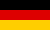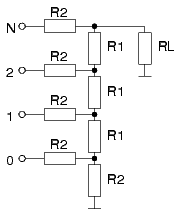# Bri's worldelectronics, programming and more

deutsch# DAC R2R network calculationThis applet calculates the output voltage of a R2R network for a digital to analog converter. The calculation includes non ideal resistors and the load at the R2R network output.
 Value R1 Value R2 Value RL Number of bits Voltage